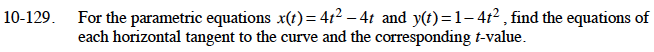### Home > CALC > Chapter Ch10 > Lesson 10.3.2 > Problem10-129

10-129.

For the parametric equations x(t) = 4t2 − 4t and y(t) = 1 − 4t2 , find the equations of each horizontal tangent to the curve and the corresponding t-value. Homework Help ✎